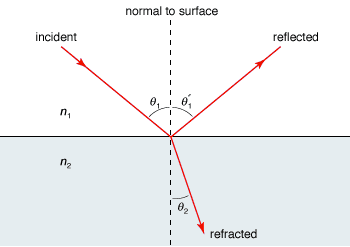# What are Difference between Reflection and Refraction of Light

Distinguish, differentiate, compare and explain what is the difference between reflection and refraction of light in physics. Comparison and Differences.

## Difference between reflection and refraction of light1. Reflection is a phenomenon when light bounces off an object or media, while refraction takes place when light bends while passing through an object.

2. In reflection, the angle of incidence is equal to the angle of reflection. In refraction angle of incidence is not equal to the angle of refraction.

3. Reflection normally occurs when the object is mirror whereas refraction prominently occurs when the object is a lens.

4. Reflection is bouncing back of light into the same medium whereas refraction is bending of light rays obliquely while passing from one medium to the other.

5. Examples of Reflection of Light: See your face in Mirror, Reflection of Light, Sound, and waves.

Examples of Refraction of Light: When there is a rainbow in the sky, the light from the sun is being refracted from the particles of water and splits the light up into different colors.

Tags:

Difference between Refraction of Light vs Reflection

Reflection vs Refraction of Light

Differences between Refraction of Light vs Reflection